# Failure Mechanics

Source: Saylor

A question pack on engineering mechanics.

Statistics Problems Q33The idealized schematic below could be thought of as an umbrella stand or a fishing rod holder. In the schematic, a narrow dowel is placed in a receptacle. The dowel is subject to a downward load FL at the outer end. FL= 50 N. We may neglect the force due to gravity acting on the dowel. The angle α = 28 degrees. The dowel is 1 meter long, and the distance d is 40 cm. The dowel is not moving. The force acting at point A is normal

A

70.26 N

B

66.22 N

C

23.47 N

D

50 N

E

93.47 N

Statistics Problems Q32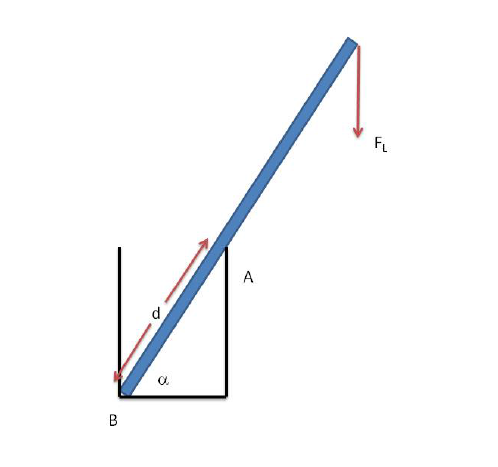The idealized schematic below could be thought of as an umbrella stand or a fishing rod holder. In the schematic, a narrow dowel is placed in a receptacle. The dowel is subject to a downward load FL at the outer end. FL= 50 N. We may neglect the force due to gravity acting on the dowel. The angle α = 28 degrees. The dowel is 1 meter long, and the distance d is 40 cm. The dowel is not moving. The force acting at point A is normal to the axis of the dowel (friction is negligible there). What is the magnitude of the force acting at point A?

A

70.26 N

B

110.37 N

C

23.47 N

D

50.00 N

E

66.22 N

Statistics Problems Q31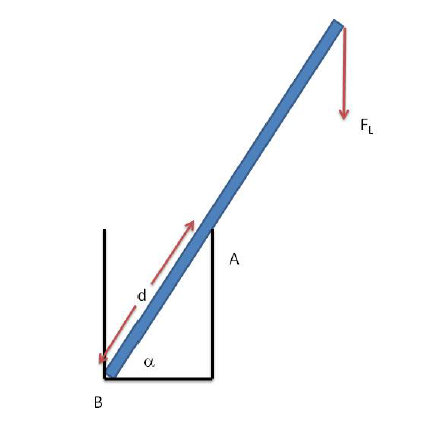The idealized schematic below could be thought of as an umbrella stand or a fishing rod holder. In the schematic, a narrow dowel is placed in a receptacle. The dowel is subject to a downward load F$_L$ at the outer end. F$_L$= 50 N. We may neglect the force due to gravity acting on the dowel. The angle α = 38 degrees. The dowel is 1 meter long, and the distance d is 40 cm. The dowel is not moving. The force acting at point A is normal to the axis of the dowel (friction is negligible there). What is the net force acting at the end of the dowel from the multiple contact points near B?

A

50.00 N

B

110.367 N

C

70.26 N

D

66.22 N

E

66.64 N

Statistics Problems Q30

Two forces acting at an angle of 60 degrees with respect to one another result in a force of 14 N. If they act at right angles, they result in a force of 11.662 N.What are the magnitudes of the two forces?

A

12 N and 7 N

B

10 N and 6 N

C

9 N and 14 N

D

15 N and 12 N

E

7 N and 8 N

Statistics Problems Q29

Two forces of magnitude 9 N and 12 N act on a point. The angle between the two forces is 30 degrees. Estimate the resultant force.

A

5 N

B

10 N

C

20 N

D

25 N

E

30 N

Statistics Problems Q28

A horizontal force is applied to a block of weight of 1500 N to hold it in position on an inclined plane which makes a 30 degree with the horizontal. Assuming no friction, calculate the horizontal force.

A

866 N

B

423 N

C

978 N

D

1232 N

E

823 N

Statistics Problems Q26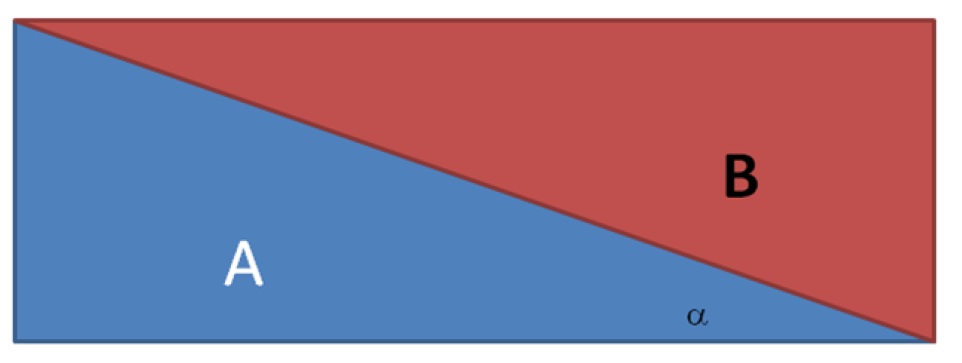In the figure below, the angle alpha is 18 degrees. The block B has a mass of 10 kg and is subject to a gravitational acceleration of 9.8 m$^2$/s straight down. Block A is being held in place by external forces.

If the coefficient of static friction is 0.05, then what is the horizontal force required to start block B moving to the left?

A

34.9 N

B

4.7 N

C

30.3 N

D

37.3 N

E

42.8 N

Statistics Problems Q27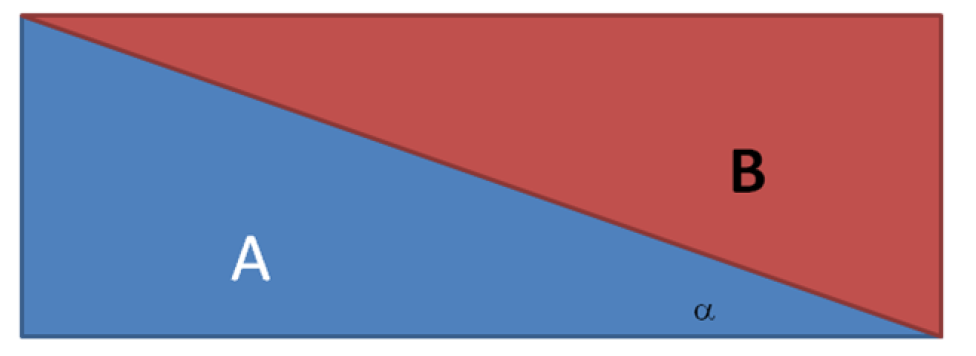In the figure below, the angle alpha is 33 degrees. The block B has a mass of 10 kg and is subject to a gravitational acceleration of 9.8 m2/s straight down. Block A is being held in place by external forces.

If the coefficient of static friction is 0.05, then what is the horizontal force required to start block B moving to the left?

A

70.8 N

B

30.7 N

C

37.4 N

D

0.57 N

E

98 N

Statistics Problems Q25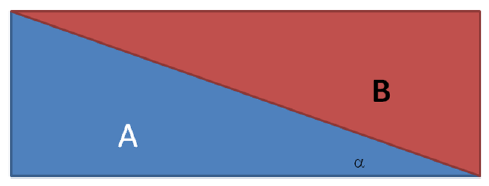In the figure below, the angle alpha is 33 degrees. The block B has a mass of 10 kg and is subject to a gravitational acceleration of 9.8 m$^2$/s straight down. Block A is being held in place by external forces.

If the coefficient of static friction is 0.07, then what is the horizontal force required to start block B moving to the left?

A

70.8 N

B

73.9 N

C

75.8 N

D

33.7 N

E

98 N

Statistics Problems Q24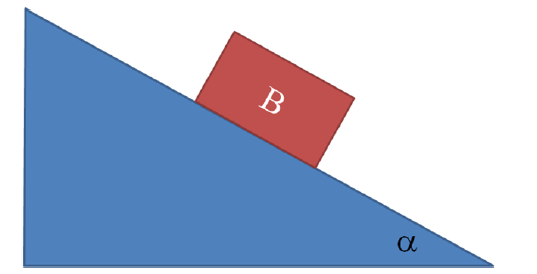As illustrated in the figure below, block B resides on an incline. The static coefficient of friction between the block and the incline is 0.343. At what value of the inclination (in degrees) will the block begin to slide under the influence of vertical gravitational acceleration?

A

21 degrees

B

2 degrees

C

19 degees

D

32 degrees

E

26 degrees

Statistics Problems Q23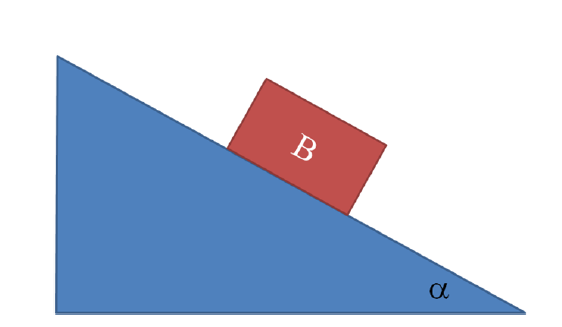As illustrated in the figure below, block B resides on an incline. The static coefficient of friction between the block and the incline is 0.38. At what value of the inclination (in degrees) will the block begin to slide under the influence of vertical gravitational acceleration?

A

21 degrees

B

2 degrees

C

7 degees

D

32 degrees

E

26 degrees

Statistics Problems Q21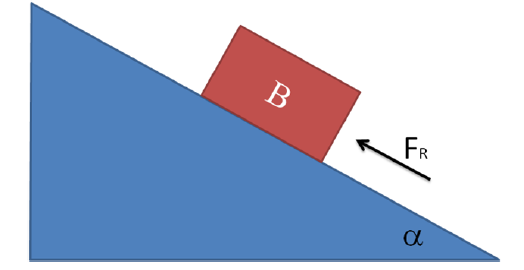In the schematic below, the block B has a mass of 25 kg. The coefficient of static friction between the block and the ramp is 0.42. The angle alpha is 0.19 radians. What is the magnitude of the force F$_R$ required to keep the block from sliding under the influence of a vertical gravitational acceleration of 9.8 m$^2$/s?

A

42 N

B

12 N

C

245 N

D

246 N

E

46 N

Statistics Problems Q22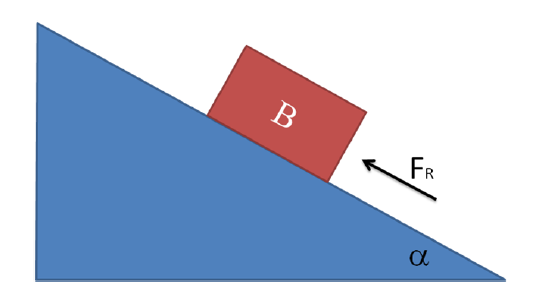In the schematic below, the block B has a mass of 18 kg. The coefficient of static friction between the block and the ramp is 0.42. The angle alpha is 0.25 radians. What is the magnitude of the force F$_R$ required to keep the block from sliding under the influence of a vertical gravitational acceleration of 9.8 m$^2$/s?

A

61 N

B

44 N

C

245 N

D

246 N

E

46 N

Statistics Problems Q20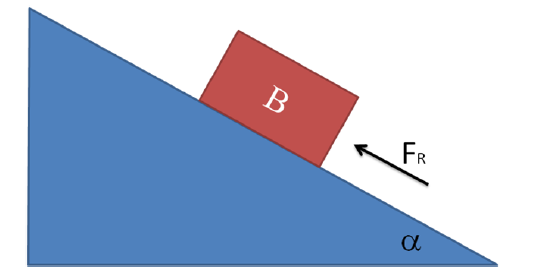In the schematic below, the block B has a mass of 25 kg. The coefficient of static friction between the block and the ramp is 0.42. The angle alpha is 0.25 radians. What is the magnitude of the force F$_R$ required to keep the block from sliding under the influence of a vertical gravitational acceleration of 9.8 m$^2$/s?

A

61 N

B

44 N

C

245 N

D

246 N

E

46 N

Statistics Problems Q19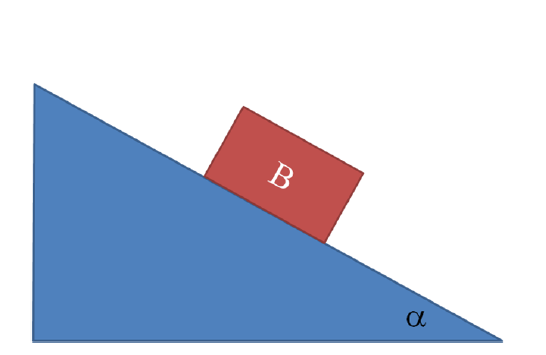As illustrated in the figure below, block B resides on an incline. The static coefficient of friction between the block and the incline is 0.488. At what value of the inclination (in degrees) will the block begin to slide under the influence of vertical gravitational acceleration?

A

21 degrees

B

2 degrees

C

19 degees

D

32 degrees

E

26 degrees

Statistics Problems Q18

The coefficient of static friction between a belt and a circular pulley is 0.62. The contact length between the belt and the pulley subtends an angle of 130 degrees. What is the ratio of belt tensions on the tight (pulling) side to the loose side of the pulley that can be accommodated without the belt slipping?

A

2.2

B

0.62

C

4.1

D

4023

E

1x10$^35$

Statistics Problems Q17

The coefficient of static friction between a belt and a circular pulley is 0.57. The contact length between the belt and the pulley subtends an angle of 150 degrees. What is the ratio of belt tensions on the tight (pulling) side to the loose side of the pulley that can be accommodated without the belt slipping?

A

4.4

B

2.6

C

1.5 x 10$^35$

D

0.57

E

7.1

Statistics Problems Q16

Consider three collinear horizontal forces acting on a rigid body. Their magnitudes are 200 N, 100 N, and 300 N. All of the forces are acting in the same direction. Determine the resultant of the forces.

A

200 N

B

300 N

C

880 N

D

600 N

E

500 N

Statistics Problems Q15

Consider three collinear horizontal forces acting on a rigid body. Their magnitudes are 20 N, 10 N, and 30 N. The force 10 N opposes the other two forces. Determine the resultant of the forces.

A

5 N

B

40 N

C

50 N

D

60 N

E

30 N

Statistics Problems Q14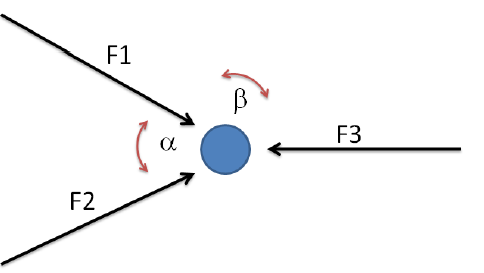In the diagram below, the angle alpha is 60 degrees and the angle beta is 150 degrees. Which of the following statements must be true in order for the particle at the center to not accelerate under the influence of forces F1, F2, and F3?

A

F1, F2, and F3 must all be of equal magnitude.

B

All forces must be zero.

C

F1 and F2 must have equal magnitude. The magnitude of F3 must be 2 cos(30 degrees) |F2|.

D

F1 and F2 must have equal magnitude. The magnitude of F3 must be 2 cos(60 degrees) |F2|.

E

F1 and F2 must have equal magnitude. The magnitude of F3 must be 2 sin(30 degrees) |F2|.

Statistics Problems Q13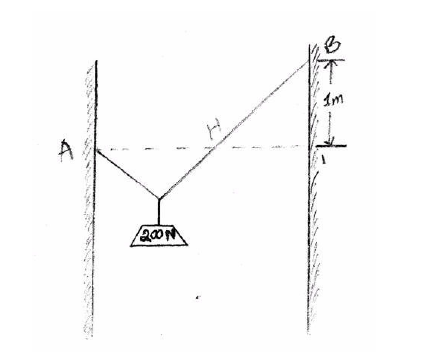Consider a rope 5 m long running from points A to B as in the figure below. The two walls are only 3 m apart; therefore, the rope sags. The difference in elevation of points A and B is 1 m. Along the rope, a frictionless pulley suspends a load of 200 N. Calculate the tension in the rope at equilibrium.

A

125 N

B

250 N

C

175 N

D

75 N

E

50 N

Statistics Problems Q12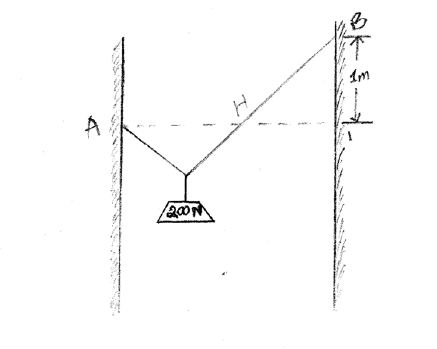Consider a rope 5 m long running from points A to B as in the figure below. The two walls are only 3 m apart; therefore, the rope sags. The difference in elevation of points A and B is 1 m. Along the rope, a frictionless pulley suspends a 200 N weight. When the system is at equilibrium, calculate the horizontal distance from the left wall to the weight.

A

0.55 m

B

1.25 m

C

1.125 m

D

2.25 m

E

0.33 m

Statistics Problems Q11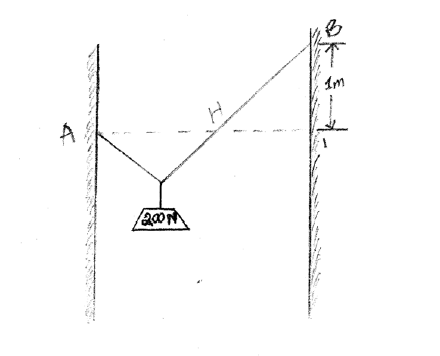Consider a rope 5 m long running from points A to B as in the figure below. The two walls are only 3 m apart; therefore, the rope sags. The difference in elevation of points A and B is 1 m. Along the rope, a frictionless pulley suspends a 200 N weight. When the system is at equilibrium, calculate the vertical distance from point A to the lowest point on the rope.

A

0.55 m

B

1.25 m

C

1.125 m

D

2.25 m

E

1.5 m

Statistics Problems Q10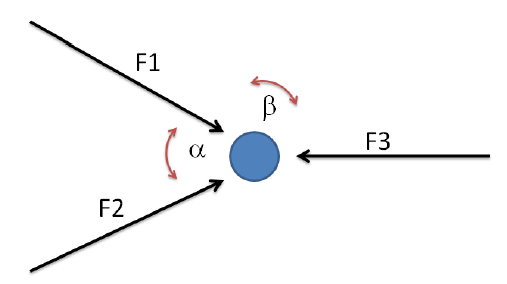In the diagram below, the angle alpha is 50 degrees and the angle beta is 155 degrees. Which of the following statements must be true in order for the particle at the center to not accelerate under the influence of forces F1, F2, and F3?

A

F1, F2, and F3 must all be of equal magnitude.

B

All forces must be zero.

C

F1 and F2 must have equal magnitude. The magnitude of F3 must be 2 cos(25 degrees) |F2|

D

F1 and F2 must have equal magnitude. The magnitude of F3 must be 2 cos(60 degrees) |F2|.

E

F1 and F2 must have equal magnitude. The magnitude of F3 must be 2 sin(30 degrees) |F2|.

Statistics Problems Q9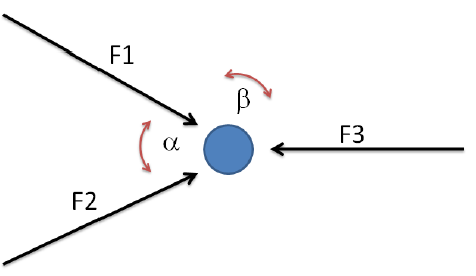In the diagram below, the angle alpha is 120 degrees and the angle beta is 120 degrees. Which of the following statements must be true in order for the particle at the center to not accelerate under the influence of forces F1, F2, and F3?

A

F1, F2, and F3 must all be of equal magnitude.

B

All forces must be zero.

C

F1 and F2 must have equal magnitude. The magnitude of F3 must be 2 cos(30 degrees) |F2|.

D

F1 and F2 must have equal magnitude. The magnitude of F3 must be 2 sin(30 degrees) |F2|.

E

F1 and F2 must have equal magnitude. The magnitude of F3 must be 2 sin(45 degrees) |F2|.

Statistics Problems Q8

The coefficient of static friction between a belt and a circular pulley is 0.57. The contact length between the belt and the pulley subtends an angle of 120 degrees. What is the ratio of belt tensions on the tight (pulling) side to the loose side of the pulley that can be accommodated without the belt slipping?

A

4.4

B

2.6

C

1.5 x 10$^{35}$

D

0.57

E

3.3

Statistics Problems Q7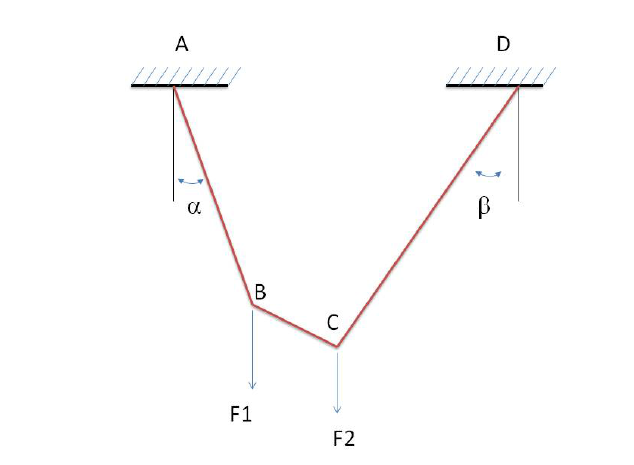Consider a wire suspended from a ceiling at points A and D and bearing two loads F1 = 20 kN and F2 = 25 kN at points C and D, respectively. The angles alpha and beta are 30 and 60 degrees, respectively. Calculate the tension in the wire segment AB.

A

190 kN

B

225 kN

C

427 kN

D

390 kN

E

475 kN

Statistics Problems Q6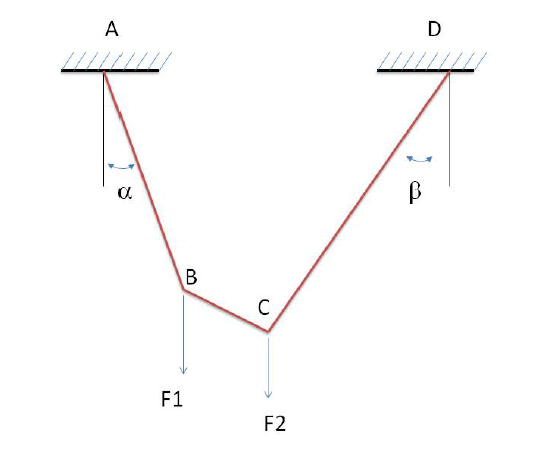Consider a wire suspended from a ceiling at points A and D and bearing two loads F1 = 20 kN and F2 = 25 kN at points C and D, respectively. The angles alpha and beta are 30 and 60 degrees, respectively. Calculate the tension in the wire segment BC.

A

190 kN

B

225 kN

C

427 kN

D

390 kN

E

475 kN

Statistics Problems Q5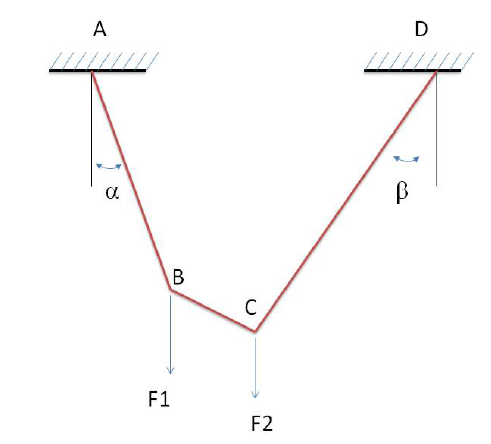Consider a wire suspended from a ceiling at points A and D and bearing two loads F1 = 20 kN and F2 = 25 kN at points C and D, respectively. The angles alpha and beta are 30 and 60 degrees, respectively. Calculate the tension in the wire segment BC.

A

190 kN

B

225 kN

C

390 kN

D

475 kN

Statistics Problems Q4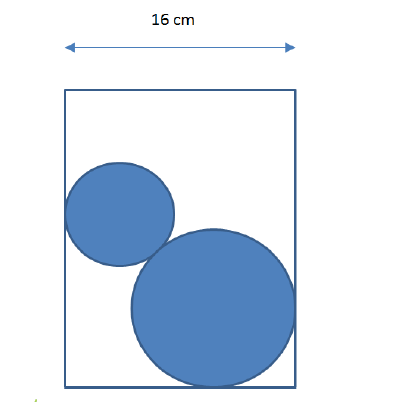Consider a circular cylinder of diameter 16 cm. The cylinder is open at both ends and placed on a smooth horizontal plane. There are 2 spheres inside the cylinder. The diameters of the spheres are 8 cm and 12 cm. The spheres weigh 10 N and 30 N respectively. Calculate the minimum weight of the cylinder so it will not tip over.

A

7.5 N

B

15 N

C

3.5 N

D

8.5 N

E

12 N

Statistics Problems Q3

A homogeneous cube of weight 100 kN sits on a horizontal plane. Determine the minimum force F acting on the upper top edge (parallel with the surface) that is required for tipping the block over.

A

100 kN

B

75 kN

C

50 kN

D

200 kN

E

25 kN

Statistics Problems Q2

Two forces of magnitude 9 N and 12 N act on a point. The angle between the two forces is 135 degrees. Estimate the resultant force.

A

5 N

B

8.5 N

C

20 N

D

17 N

E

30 N

Statistics Problems Q1

Two forces of magnitude 9 N and 12 N act on a point. The angle between the two forces is 75 degrees. Estimate the resultant force.

A

5 N

B

8.5 N

C

20 N

D

17 N

E

30 N

CC BY 3.0 - Saylor - Steve Gibbs